### Home > AC > Chapter 14 > Lesson 14.3.1.2 > Problem3-26

3-26.

Each table below represents an exponential function of the form y = abx. Copy and complete each table on your paper and find the corresponding equation. Homework Help ✎

1.  x y 0 1 2 3 4 1.8 5.76 18.432
2.  x y 0 1 2 3 4 5 245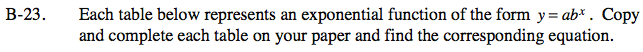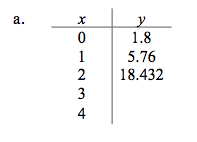What is the multiplier?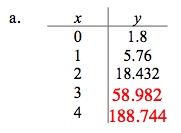y = 1.8(3.2)x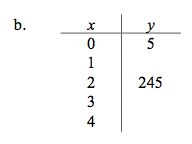5 · m · m = 245
Solve for m.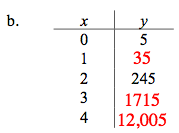y = 5(7)x